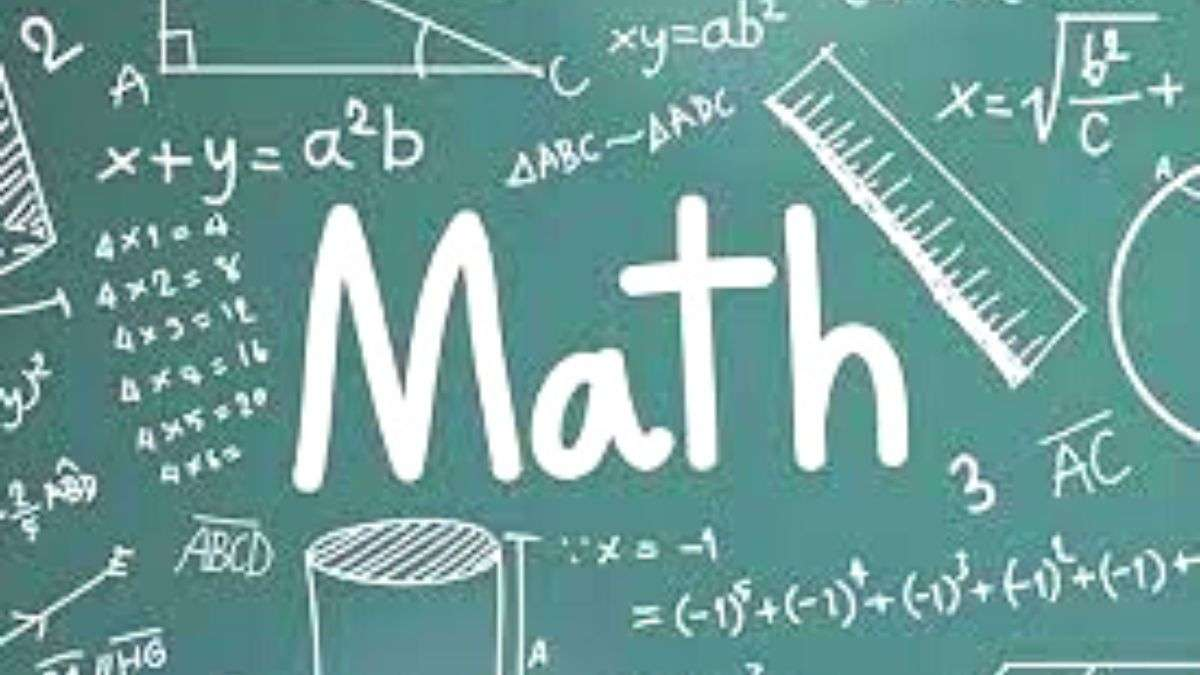# Math riddles with answers: Math is tough, but fun too! Let these math riddles prove to you how fun math can be!

When was the last time you picked your school math book or chose to solve heavy calculations yourself without the use of a calculator? Well, these math riddles will take you back to those times.“Math is a super easy subject that anyone can master”, said no man ever.

Math is indeed challenging, but it can never be boring. The complex theorems, bind-twisting numbers, and complicated algebraic expressions; all conspire to make you stay awake when you feel drowsy. A popular trick to staying awake when you feel extremely sleepy is to calculate a complex math problem in the mind. Moreover, according to some human psychology beliefs, the same trick can be applied to cases when you wish to control your laughter in times of odd situations. Yes, math is genuinely useful in everyday life.

Feeling bored? Let us bring math to your rescue! These math riddles will surely add some moments of fun to your day. Are you ready?

## MATH RIDDLE 1:

Once there was a lobster who was very fond of studies. He went to the best school in the woods. What do you think he got on the mathematics test?

## Math Riddle 2:

X is a magical number. Add, subtract, or multiply it with any number, and no change will happen. Do you know “X”?

## Math Riddle 3:

Which of the following is correct?

• Eighty-seven plus fifty-three is One hundred and thirty-nine.
• Eighty-seven plus fifty-three are One hundred and thirty-nine.

Brain Teasers: How good were you at geography? Let’s find out today!

If 1=3

2=3

3=5

4=4

5=4

Then, 6=?

## Math Riddle 5:

I am an odd number. Take away one letter and I become even. What number am I?

MATH RIDDLE 1:

Once there was a lobster who was very fond of studies. He went to the best school in the woods. What do you think he got on the mathematics test?

The lobster got a Sea-plus on the math test. Don't you think he needs improvement?

Math Riddle 2:

X is a magical number. Add, subtract, or multiply it with any number, and no change will happen. Do you know “X”?

The magical number X is 0.

Math Riddle 3:

Which of the following is correct?

• Eighty-seven plus fifty-three is One hundred and thirty-nine.
• Eighty-seven plus fifty-three are One hundred and thirty-nine.

None.

Forget subject-verb agreement, 87 + 53 = 140!

Math Riddle 4:

If 1=3

2=3

3=5

4=4

5=4

Then, 6=?

3, as ‘six’ has three letters

Math Riddle 5:

I am an odd number. Take away one letter and I become even. What number am I?